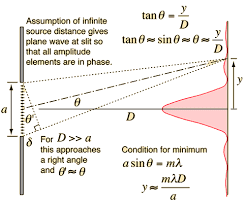# How is the amplitude of a wave related to the slit width?

Faiq

## Homework Statement

How is the amplitude of a wave affected if the slit separation remains same but slit width is
1.)Increased?
2.)Decreased?
and why?

## Homework Equations

Intensity is proportional to the square of amplitude

## The Attempt at a Solution

I consider the amplitude as allowed "fatness" of a wave. The more slit width there is, the more wave can be "fat" and so it will have a greater amplitude.

gracy
As you wrote intensity is proportional to the square of amplitude.
Do you know the relation between intensity of wave and the width of slits?
The intensity of light due to a slit (source of light) is directly proportional to width of the slit.
Therefore if the two slits ##S_1## & ##S_2## have widths ##W_1## & ##W_2## respectively and ##I_1## & ##I_2## are intensities of light waves having amplitude ##a_1## and ##a_2## from respective slits then,

##\frac{I_1}{1_2}## ∝ ##\frac{a_1^2}{a_2^2}## ∝ ##\frac{W_1}{W_2}##

Faiq
Yes, I know that. I am asking why does the amplitude increases with increasing slit width

gracy
Yes, I know that. I am asking why does the amplitude increases with increasing slit width
In single slit diffraction calculations, the resultant amplitude is obtained by dividing the slit width into a large number of equal segments. For each segment, the amplitude is taken proportionally equal and the resultant amplitude is found by superposition of amplitudes of all the segments at the point of consideration.
So amplitude should be proportional to slit width.

gracyCan you see division of the slit width into a large number of equal segments?

gracy

ube
Then (amplitude)^2 is proportional to slit width or it is amplitude proportional to slit width??

ube
The amplitude of the wave passing through a slit is directly proportional to its width. If the slit width is x and amplitude of the wave is A, then A ∝ x.

Homework Helper
Gold Member
2022 Award
HI @ube. For information, you are replying to a very old (April 2016) thread.

Note, the original question is unclear/incomplete. The Homework Statement refers to 'slit separation' - I would guess the question is actually about the intensity of the central maximum in a Young's double slit experiment.

•